• 局部二值模式直方图
万次阅读
2018-08-07 10:04:22
#include <opencv2/core.hpp>
#include <opencv2/face.hpp>
#include <opencv2/highgui.hpp>

#include <iostream>
#include <fstream>
#include <sstream>

using namespace std;
using namespace cv;
using namespace cv::face;

void read_csv(string& filename, vector<Mat>& images, vector<int>& labels, char separator = ';')
{
ifstream file;
file.open(filename.c_str(), ifstream::in);
if (!file)
{
printf("can't open file: %s\n", filename.c_str());
exit(-1);
}

string line, path, classlabel;
while (getline(file, line))
{
stringstream liness(line);
getline(liness, path, separator);
getline(liness, classlabel);
if (!path.empty() && !classlabel.empty())
{
labels.push_back(atoi(classlabel.c_str()));
}
}
}

int main()
{
string filename = string(".\\face_recg\\face\\at.txt");

vector<Mat> images;
vector<int> labels;

try
{
}
catch (const cv::Exception& e)
{
printf("failed opening file %s reason %s\n", filename.c_str(), e.msg.c_str());
exit(1);
}

if (images.size() <= 1)
{
printf("This demo needs at least 2 images to work. Please add more images to your data set!\n");
exit(1);
}

Mat testSample = images[images.size() - 1];
int testLabel = labels[labels.size() - 1];

images.pop_back();
labels.pop_back();

Ptr<LBPHFaceRecognizer> model = LBPHFaceRecognizer::create();
model->train(images, labels);

int predictLabel = model->predict(testSample);

printf("test label = %d, predict label = %d\n", testLabel, predictLabel);

//model->setThreshold(0.0);
//predictLabel = model->predict(testSample);

//printf("test label = %d, predict label = %d\n", testLabel, predictLabel);

//打印参数
int neibs = model->getNeighbors();	//周围像素点的个数
int grid_x = model->getGridX();		//将一张图片在x方向分成几块
int grid_y = model->getGridY();		//将一张图片在y方向分成几块
double threshold = model->getThreshold();	//相似度阈值

printf("neibs = %d\n", neibs);
printf("grid_x = %d\n", grid_x);
printf("grid_y = %d\n", grid_y);
printf("threshold = %e\n", threshold);

vector<Mat> histograms = model->getHistograms();
printf("Size of the histograms: %zd\n", histograms.total());

waitKey(0);

return 0;
}openCV
更多相关内容
• 先把代码搁这，纪录一下，可以直接运行摄像头检测： import os import cv2 import numpy as np from sklearn import preprocessing # Class to handle tasks related to label encoding class LabelEncoder...

先把代码搁这，纪录一下，可以直接运行摄像头检测：


import os

import cv2
import numpy as np
from sklearn import preprocessing

# Class to handle tasks related to label encoding
class LabelEncoder(object):
# Method to encode labels from words to numbers
def encode_labels(self, label_words):
self.le = preprocessing.LabelEncoder()
self.le.fit(label_words)

# Convert input label from word to number
def word_to_num(self, label_word):
return int(self.le.transform([label_word]))

# Convert input label from number to word
def num_to_word(self, label_num):
return self.le.inverse_transform([label_num])

# Extract images and labels from input path
def get_images_and_labels(input_path):
label_words = []

# Iterate through the input path and append files
for root, dirs, files in os.walk(input_path):
for filename in (x for x in files if x.endswith('.jpg')):
filepath = os.path.join(root, filename)
label_words.append(filepath.split('\\')[-2])

# Initialize variables
images = []
le = LabelEncoder()
le.encode_labels(label_words)
labels = []

# Parse the input directory
for root, dirs, files in os.walk(input_path):
for filename in (x for x in files if x.endswith('.jpg')):
filepath = os.path.join(root, filename)

# Read the image in grayscale format
image = cv2.imread(filepath, 0)

# Extract the label
name = filepath.split('\\')[-2]

# Perform face detection
faces = faceCascade.detectMultiScale(image, 1.1, 2, minSize=(100,100))

# Iterate through face rectangles
for (x, y, w, h) in faces:
images.append(image[y:y+h, x:x+w])
labels.append(le.word_to_num(name))

return images, labels, le

if __name__=='__main__':

# Initialize Local Binary Patterns Histogram face recognizer
recognizer = cv2.face.LBPHFaceRecognizer_create()

# Extract images, labels, and label encoder from training dataset
images, labels, le = get_images_and_labels(path_train)

# Train the face recognizer
print( "\nTraining...")
recognizer.train(images, np.array(labels))
# Test the recognizer on unknown images
print( '\nPerforming prediction on test images...')
cap = cv2.VideoCapture(0)

# Define the scaling factor

# Loop until you hit the Esc key
while True:
# Capture the current frame and resize it
ret, frame = cap.read()
frame1=cv2.resize(frame, None, fx=0.5, fy=0.5,interpolation=cv2.INTER_AREA)
gray = cv2.cvtColor(frame1, cv2.COLOR_BGR2GRAY)
#predict_image = cv2.resize(frame, None, fx=scaling_factor, fy=scaling_factor,interpolation=cv2.INTER_AREA)
faces = faceCascade.detectMultiScale(gray, 1.1,2, minSize=(100,100))
# Convert to grayscale
#gray = cv2.cvtColor(frame, cv2.COLOR_BGR2GRAY)

# Run the face detector on the grayscale image
#face_rects = face_cascade.detectMultiScale(gray, 1.3, 5)

# Draw rectangles on the image
for (x, y, w, h) in faces:
# Predict the output
predicted_index, conf = recognizer.predict( gray[y:y+h, x:x+w])

# Convert to word label
predicted_person = le.num_to_word(predicted_index)

# Overlay text on the output image and display it
cv2.putText(frame1, predicted_person,   (10,60), cv2.FONT_HERSHEY_SIMPLEX, 2, (255,255,255), 3)
cv2.imshow("Recognizing face", frame1)

c = cv2.waitKey(5)
if c == 27:
break
# Check if Esc key has been pressed

# Release the video capture object and close all windows
cap.release()
cv2.destroyAllWindows()




展开全文• 一）局部二值模式简介 前面介绍过关于主成分分析法的识别方法，这个方法是选取的整幅图像的主成分分量来作为新的特征，从某种意义上说这是一种基于全局特征的方法。但是一般图像识别中，仅仅使用全局特征是不够...

一）局部二值模式简介

前面介绍过关于主成分分析法的识别方法，这个方法是选取的整幅图像的主成分分量来作为新的特征，从某种意义上说这是一种基于全局特征的方法。但是一般图像识别中，仅仅使用全局特征是不够的，获得的识别率较低，更多的时候，表征一副图像的特征也缺少不了局部特征。那么局部二值模式（LBP）就是这样一种表征局部特征的方法。

介绍性原理的文章很多，这里贴个较好的：

研究该算法的过程中还发现了一篇较好的硕士论文：

基于LBP的人脸识别研究_黄非非

想深入了解的可以看看。

一）经典LBP特征提取的程序实现

在介绍实现之前请一定要看上述的那篇博客，那个里面有相关的介绍原理，看了这个原理才能知道算法的实现过程。这里也是介绍经典的矩形3*3领域实现方法。

从上述原理可以知道，要得到图像中的每个像素点的LBP值，必须和它的领域有关，所以它的四周必须得有像素点，这就出现了一个问题，对于一个图像边缘像素点来说怎么办？它是边缘点，那么必定存在着它的3*3领域内的某几个点超出图像之外，也就是没有像素值，这样会出现错误的，那么一个解决办法就是对原图像进行像素扩展，也就是把原图像的四周向外扩展一下，像素点的灰度扩展成多少？可以是把边界点的像素复制过去就可以了（当然还有其他方法），知道图像滤波的可能会知道，在滤波算法中也存在着边界点的处理过程，本质上方法是一样的。

考虑到是3*3的矩形领域，那么四周边界点只需要向外扩展一行或者一列就行了。如果矩形框改变大小，那么向外扩展的大小也改变。简单的扩展描述过程如下：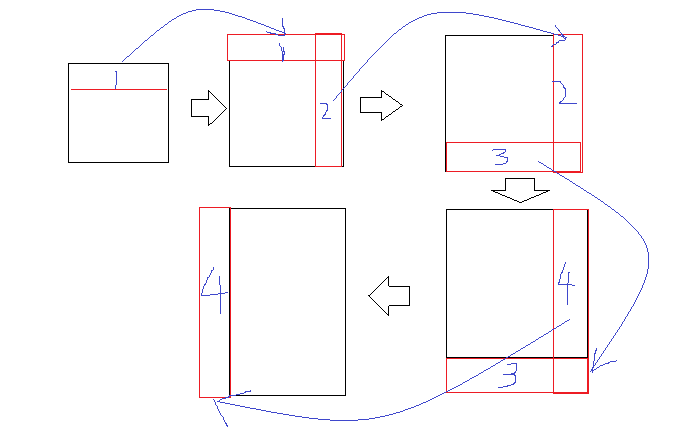把相应的位置复制到新的扩展图中就可以了。

从原理中看到某个像素点的LBP值得计算公式可以简单描述为：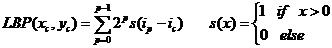其中，2^p的系数可以用矩阵代替：C = [1,2,4;128,0,8;64,32,16];

求和可以用矩阵的点乘来代替并求和。矩阵点乘就是矩阵对应位置与对应位置相乘，和矩阵直接相乘还是有很大的区别。

有了上述分析，程序可以写成如下：

%--------------函数说明-------------
%-----LBP：Local Binary Patterns，局部二值模式
%-----输入:img原始灰度图像:注意图片为double型
%-----输出:该图像的的LBP特征
%-----------------------------------
function LBP = Lbp_Img(img)
%% %%%%%%%%%%%%%%%%%%%%%%%%%%%%%%%%%%%
[m,n] = size(img);%取大小
r = 1;
img_extend = zeros(m+2*r,n+2*r);%规定扩展后的矩阵大小并赋值0
img_extend(r+1:m+r,r+1:n+r) = img;%中间部分复制
img_extend(1:r,r+1:n+r) = img(1:r,1:n);%上扩展
img_extend(1:m+r,n+r+1:n+2*r) = img_extend(1:m+r,n+1:n+r);%右扩展
img_extend(m+r+1:m+2*r,r+1:n+2*r) = img_extend(m+1:m+r,r+1:n+2*r);%下扩展
img_extend(1:m+2*r,1:r) = img_extend(1:m+2*r,r+1:r+1:2*r);%左扩展

%%
C = [1,2,4;128,0,8;64,32,16]; %矩阵点乘的权值矩阵
LBP = zeros(m,n);
for i=1:m   %对所有的像素点
for j=1:n
temp = img(i,j);   %取得该点像素值--也就是中心值
temp_mat = ones(3)*double(temp);  %检测得邻域矩阵3*3
temp_ext = img_extend(i+r-1:i+r+1,j+r-1:j+r+1);%取得邻域矩阵像素点
temp_mean = temp_ext - temp_mat;  %相差
temp_mean(temp_mean>=0) = 1; %判断，置0与1
temp_mean(temp_mean<0) = 0;
LBP(i,j) = sum(sum(C.*temp_mean));   %点乘并求和返回LBP
end
end


文件夹名字为Lbp_Img.m

二）:样本图片的LBP提取与直方图显示：

现在对一副图像进行提取整个图像的LBP,很显然一幅图像的LBP必定是与原图像相同大小的矩阵才对。现在我们随意找来一副图像进行提取LBP：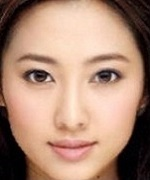首先转化成灰度图像，并进行double型转换，最后直接运行

src=imread('F:\ZQX\32x256.bmp');
I=rgb2gray(src);
LBP = Lbp_Img(I);
imshow(LBP,[]);%这个地方需要加[]，自适应的显示


文件夹名字为：test.m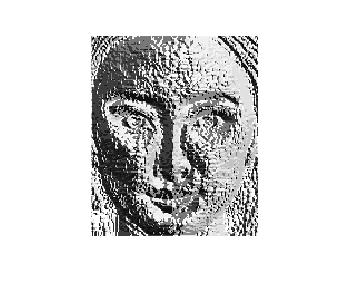LBP算法的一大优点就是对光照具有较强的鲁棒性，现在人为用matlab改变一下样本的原始亮度，再显示他们的LBP如下所示：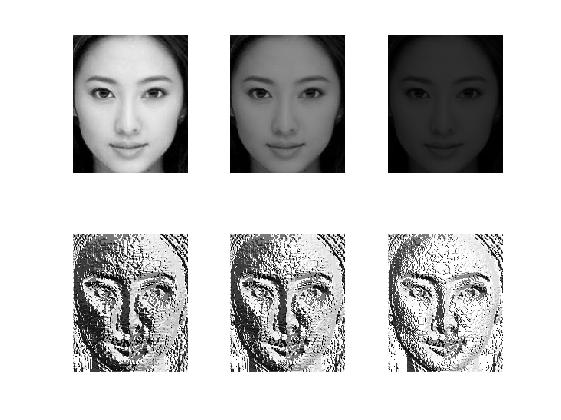可以看到在亮度不同的条件下他们的LBP基本上没有多大变化。

那么怎么去进行后续处理呢？一个很重要的处理方法就是对LBP进行灰度直方图处理，在直方图的基础上进一步处理。

对上述得到的LBP，matlab本身自带直方图统计与显示函数hist，但是它只能处理一行或者一列的数据，所以对LBP这种矩阵首先得进行处理成一行再来运用，这里我们又可以运用reshape函数了。处理与显示如下：

[m,n] = size(LBP);
data = reshape(LBP,1,m*n);
hist(data,255);
[nel,cen] = hist(data,255);


统计直方图如下所示：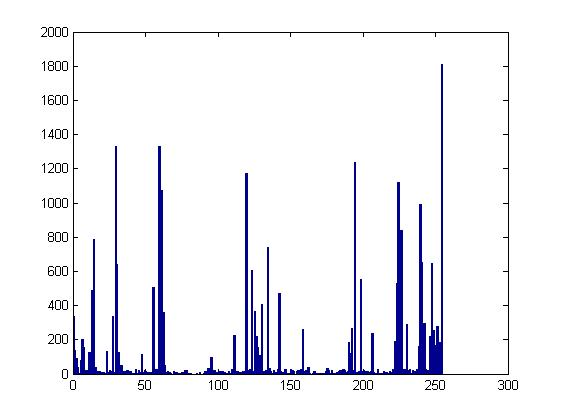还有一个我们关心的数字就是上述的nel，这是每个像素点的LBP中的总统计个数,而cen是中心点，其实就是1~255（所有灰度值）。nel这个1*n的数据在后面的处理时至关重要。

最后自己画一个图 帮助理解：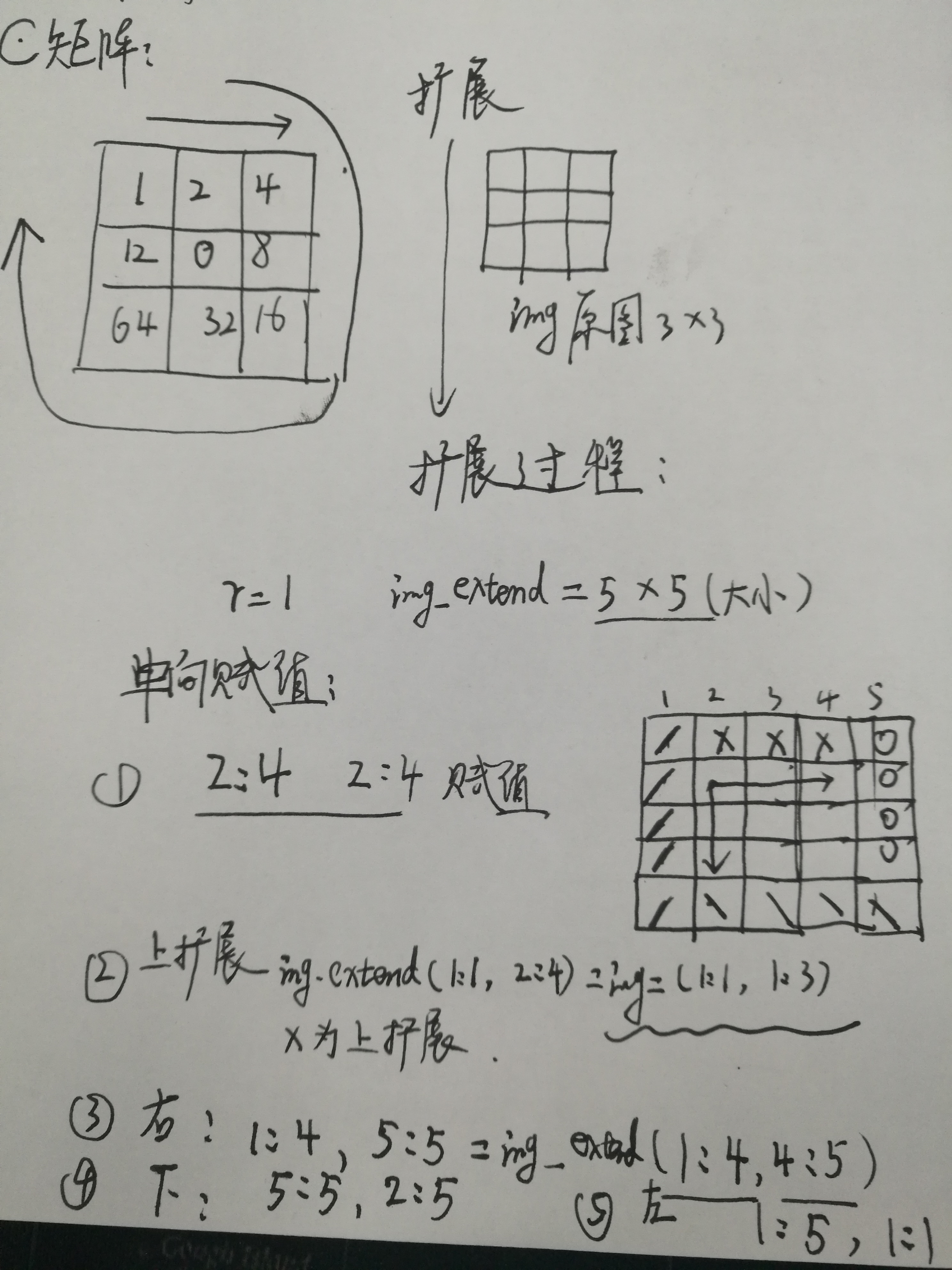展开全文• 图像块的 LBP（直方图和图像）。 旋转不变并有统一选项。 统一 - 顶部有 2 个过渡的矢量示例：00000000（0 次转换） ... 在 NOTuniform 中，我们有 2^8 个选项，向量将拆分为所有选项，因此我们希望直方图中的较低。matlab
• 基于这些自适应局部二值模式，我们进一步提出了以邻域直方图策略对图像表示的微结构进行编码的方法。对四个医学数据集进行了广泛的自评估，表明该方法显着改善了标准LBP，并与其他几种流行方法相比具有优势。
• 简要介绍LBP（Local Binary Pattern，局部二值模式）是一种用来描述图像局部纹理特征的算子，具有旋转不变性和灰度不变性等显著优点。首先由T. Ojala, M.Pietikäinen, 和D. Harwood 在1994年提出，用于纹理特征提取...

### 简要介绍

LBP（Local Binary Pattern，局部二值模式）是一种用来描述图像局部纹理特征的算子，具有旋转不变性和灰度不变性等显著优点。首先由T. Ojala, M.Pietikäinen, 和D. Harwood 在1994年提出，用于纹理特征提取。主要应用于人脸识别和目标检测中，OpenCV中有使用LBP特征进行人脸识别的接口，也有用LBP特征训练目标检测分类器的方法，Opencv实现了LBP特征的计算，但没有提供一个单独的计算LBP特征的接口。

### 原始LBP

3×3 $3 \times 3$ 的窗口中，以窗口中心像素为阈值，将相邻的8个像素的灰度值与其进行比较，若周围像素值大于中心像素值，则该像素点的位置被标记为1，否则为0。可以产生8位二进制数，将其转化为十进制数便得到了LBP编码（256种），如下图左上角开始遍历组成2进制数，转化为10进制后为124。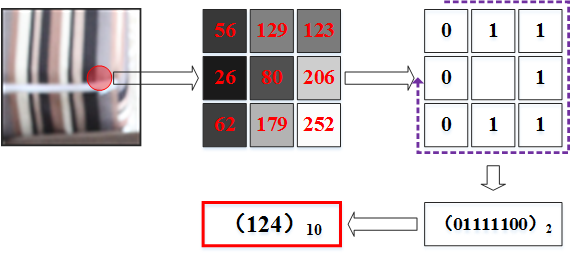具体的，其数学表达可写作：

LBP(xc,yc)=p=0p12ps(ipic)

其中， (xc,yc) $(x_c,y_c)$为中心像素的坐标， p $p$为邻域的第p个像素，ip$i_p$为邻域像素的灰度值， ic $i_c$为中心像素的灰度值， s(x) $s(x)$为符号函数，如下：
s(x)={1,0,x>=0x<0

代码实现如下：

void orLBP(Mat& src, Mat &dst)
{
// 循环处理图像数据
for (int i = 1; i < src.rows - 1; i++)
{
for (int j = 1; j < src.cols - 1; j++)
{
uchar center = src.at<uchar>(i, j);
unsigned char lbpCode = 0;
lbpCode |= (src.at<uchar>(i - 1, j - 1) > center) << 7;
lbpCode |= (src.at<uchar>(i - 1, j) > center) << 6;
lbpCode |= (src.at<uchar>(i - 1, j + 1) > center) << 5;
lbpCode |= (src.at<uchar>(i, j + 1) > center) << 4;
lbpCode |= (src.at<uchar>(i + 1, j + 1) > center) << 3;
lbpCode |= (src.at<uchar>(i + 1, j) > center) << 2;
lbpCode |= (src.at<uchar>(i + 1, j - 1) > center) << 1;
lbpCode |= (src.at<uchar>(i, j - 1) > center) << 0;
dst.at<uchar>(i - 1, j - 1) = lbpCode;
}
}
}

int main()
{
int radius = 1;
imshow("原始图像", img);

//创建一个LBP，注意为了防止溢出，我们行列都在原有图像上减去2个半径
Mat dst = Mat(img.rows - 2 * radius , img.cols - 2 * radius , CV_8UC1, Scalar(0));
orLBP(img, dst);
imshow("原始LBP", dst);
cv::waitKey(0);
}

不足：只覆盖了一个固定半径范围内的小区域，不能满足不同尺寸和频率纹理的需要。

### 圆形LBP

为适应不同尺度的纹理特征，并达到灰度和旋转不变性的要求，Ojala等做出了改进，将 3×3邻域扩展到任意邻域，并用圆形邻域代替正方形邻域，改进后的 LBP 算子允许在半径为 R 的圆形邻域内有任意多个像素点。从而得到了诸如半径为R的圆形区域内含有P个采样点的LBP算子。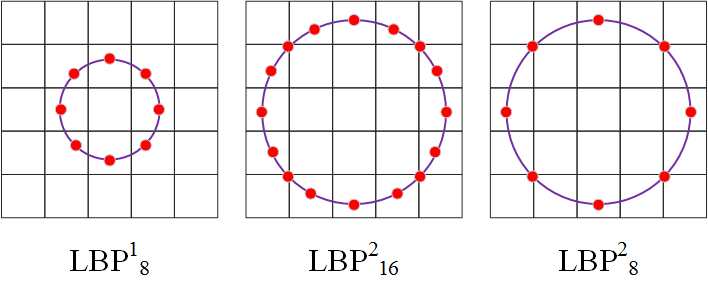其中， LBPrp $LBP_p^r$表示在以 r $r$为半径的圆形领域内，含有p$p$个采样点的LBP局部编码。这种LBP特征叫做Extended LBP，也叫Circular LBP，使用可变半径的圆对近邻像素进行编码，可以得到诸如下面的近邻描述（Spot / Line / Corner / Edge ) 。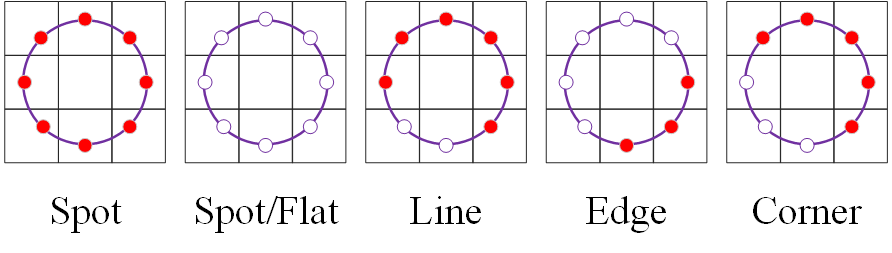要比较中心像素与周围领域的大小，首先要知道领域内点的坐标在哪吧，那周围这些点怎么确定呢？对于给定中心点 (xc,yc) $(x_c,y_c)$，其邻域像素位置为 (xp,yp)pP $(x_p,y_p)，p \in P$，其采样点 (xp,yp) $(x_p,y_p)$用如下公式计算：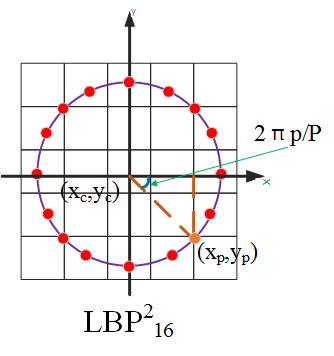xp=xc+Rcos(2pπP)yp=ycRsin(2pπP)

R是采样半径，p是第p个采样点，P是采样数目。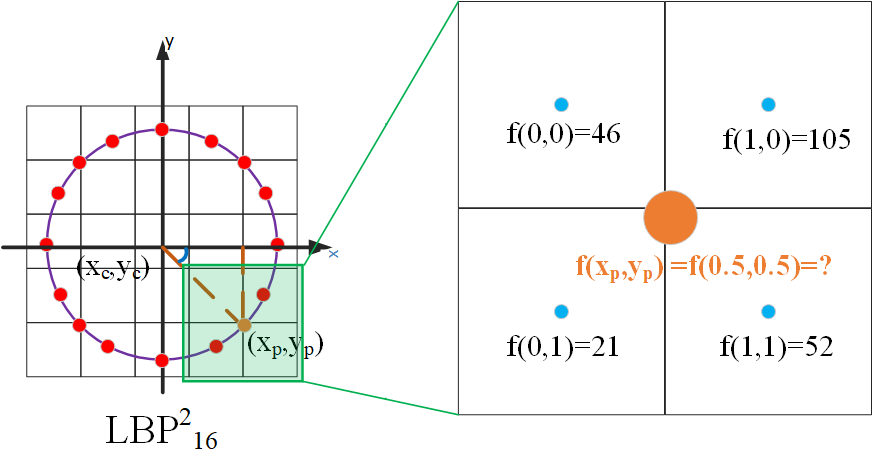糟糕的是，我们将计算的坐标位置局部放大来看，并假设计算出的位置周围的图像坐标分别是从 (0,0)(1,1) $(0,0)\rightarrow(1,1)$，我们计算出来的坐标并不一定是整数，即计算出来的点不在图像上，怎么确定他的像素值呢？或者说他的像素值取多少比较合理？这种情况下，我们一般采用插值计算算出它的合理的像素值，目前的插值方法有很多，Opencv使用的是双线性插值，双线性插值的公式如下：

f(x,y)[1xx][f(0,0)f(1,0)f(0,1)f(1,1)][1yy]

或写作权重的形式如下：
f(P)=f(x,y)f(0,0)(1x)(1y)+f(1,0)x(1y)+f(0,1)(1x)y+f(1,1)xy

对该公式不理解的可以参考我的博客 (双线性插值)。这里通过一个偏移，可以选择一个坐标系统使得f的四个已知点坐标分别为 (0, 0)、(0, 1)、(1, 0) 和 (1, 1)，P(x,y)是需要插值的点。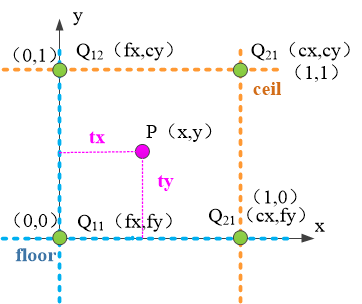因此，按照上述分析，在C++中圆形LBP实现代码如下：

void elbp(Mat& src, Mat &dst, int radius, int neighbors)
{

for (int n = 0; n < neighbors; n++)
{
// 采样点的计算
float x = static_cast<float>(-radius * sin(2.0*CV_PI*n / static_cast<float>(neighbors)));
float y = static_cast<float>(radius * cos(2.0*CV_PI*n / static_cast<float>(neighbors)));

// 上取整和下取整的值
int fx = static_cast<int>(floor(x));
int fy = static_cast<int>(floor(y));
int cx = static_cast<int>(ceil(x));
int cy = static_cast<int>(ceil(y));

// 小数部分
float ty = y - fy;
float tx = x - fx;

// 设置插值权重
float w1 = (1 - tx) * (1 - ty);
float w2 = tx  * (1 - ty);
float w3 = (1 - tx) *      ty;
float w4 = tx  *      ty;

// 循环处理图像数据
for (int i = radius; i < src.rows - radius; i++)
{
for (int j = radius; j < src.cols - radius; j++)
{
// 计算插值
float t = static_cast<float>(w1*src.at<uchar>(i + fy, j + fx) + w2*src.at<uchar>(i + fy, j + cx) + w3*src.at<uchar>(i + cy, j + fx) + w4*src.at<uchar>(i + cy, j + cx));
// 进行编码
dst.at<uchar>(i - radius, j - radius) += ((t > src.at<uchar>(i, j)) || (std::abs(t - src.at<uchar>(i, j)) < std::numeric_limits<float>::epsilon())) << n;
}
}
}
}

### 旋转不变LBP

上述LBP特征已具有灰度、光照不变性，但还不具备旋转不变性，为了实现旋转不变性，研究人员又提出了具有旋转不变性的LBP特征。 其主要思想大致是：首先不断的旋转圆形邻域内的LBP特征，根据选择得到一系列的LBP特征值，从这些LBP特征值选择LBP特征值最小的作为中心像素点的LBP特征。具体做法如下图所示：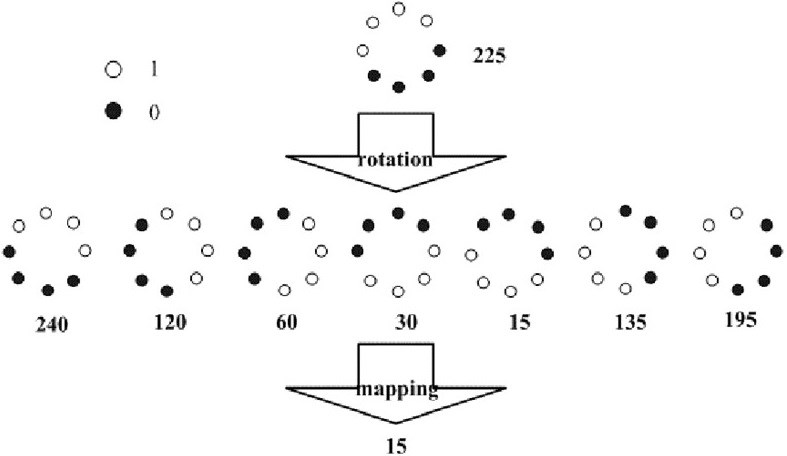如图，通过对得到的LBP特征进行旋转，得到一系列的LBP特征值，最终将特征值最小的一个特征模式作为中心像素点的LBP特征。其实现的关键代码如下：

//进行旋转不变处理
for (int i = 0; i<dst.rows; i++)
{
for (int j = 0; j<dst.cols; j++)
{
unsigned char currentValue = dst.at<uchar>(i, j);
unsigned char minValue = currentValue;
for (int k = 1; k<neighbors; k++)
{
//循环左移
unsigned char temp = (currentValue >> (neighbors - k)) | (currentValue << k);
if (temp < minValue)
{
minValue = temp;
}
}
dst.at<uchar>(i, j) = minValue;
}
}`

### 等价模式（Uniform Pattern）

上述LBP算子可以产生各种不同的二进制模式，对于半径为R圆形区域内含有P个采样点的LBP算子将会产生 2P $2^P$种模式。很显然，随着邻域集内采样点数的增加，二进制模式的种类是急剧增加的。例如：5×5邻域内20个采样点，有 2201,048,576 $2^{20}＝1,048,576$种二进制模式。如此多的模式无论对于纹理提取还是对于纹理识别、分类及信息存取都是不利的。同时，过多的模式种类对于纹理的表达是不利的。例如，将LBP算子用于纹理分类或人脸识别时，常采用LBP模式的统计直方图来表达图像的信息，而较多的模式种类将使得数据量过大，且直方图过于稀疏。因此，需要对原始的LBP模式进行降维，使得数据量减少的情况下能最好的代表图像的信息。

那么如何解决二进制模式过多的问题，对LBP算子进行降维呢？Ojala采用一种“等价模式”(Uniform Pattern)来对LBP算子进行降维。他认为在实际图像中，绝大多数LBP模式最多只包含两次从1到0或从0到1的跳变。因此，Ojala将“等价模式”定义为：

当某个LBP所对应的循环二进制数从0到1或从1到0最多有两次跳变时，该LBP所对应的二进制就称为一个等价模式类。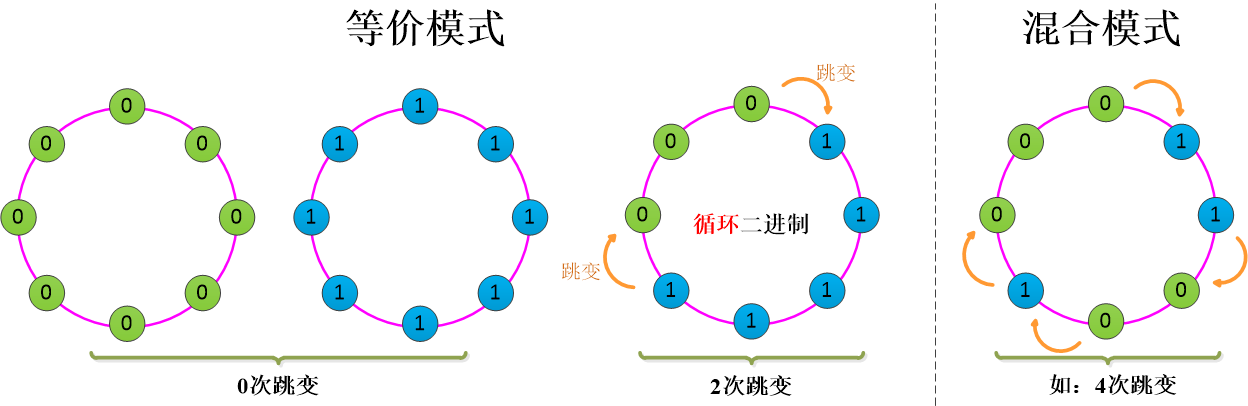如上述左边，0次跳变和2次跳变为等价模式，除此之外其他模式的都是混合模式。那这种LBP模式与传统的LBP有何区别和联系呢？采样点数目为8个，即LBP特征值有 28 $2^8$种，共256个值，正好对应灰度图像的0-255，因此原始的LBP特征图像是一幅正常的灰度图像，而等价模式LBP特征，根据0-1跳变次数，将这256个LBP特征值分为了59类，从跳变次数上划分如下表所示（ 需要注意的是不可能出现奇数次的跳变，因为有一次 01 $0 \rightarrow 1$，就相应的应该有一次 10 $1 \rightarrow 0$，因为它是循环的。）：

跳变次数模式个数跳变次数模式个数
0210
25630
414050
65670
82

共9种跳变情况，将这256个值进行分配，跳变小于2次的为等价模式类，共58个，他们对应的值按照从小到大分别编码为1—58，即它们在LBP特征图像中的灰度值为1—58，而除了等价模式类之外的混合模式类被编码为0，即它们在LBP特征中的灰度值为0，因此等价模式LBP特征图像整体偏暗。

好了，现在通过这样的改进，二进制模式的种类大大减少，而不会丢失任何信息。模式数量由原来的 2P $2^P$种减少为 P(P1)+2 $P ( P-1)+2$种，其中P表示邻域集内的采样点数。对于3×3邻域内8个采样点来说，二进制模式由原始的256种减少为58种，即：它把值分为59类，58个uniform pattern为一类，其它的所有值为第59类。这样直方图从原来的256维变成59维。这使得特征向量的维数更少，并且可以减少高频噪声带来的影响。 现在解释下：

模式数量： 2P $2^P$ P(P1)+3 $\rightarrow P ( P-1)+3$

很显然，在上面的图中叙述的很清楚，等价模式中0次跳变共两种，还要加一个混合模式，这是为什么在后面加3的原因，那么 P(P1) $P(P-1)$呢，且看下图，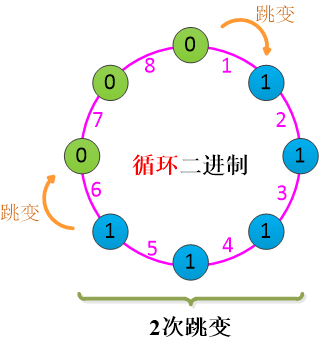在2次跳变中，紫色的数字表示跳变的位置，假设有P个采样点，首先从P个位置中选一个 01 $0 \rightarrow 1$的跳变，即有 C1P $C_P^1$种选择，紧接着在剩下的P-1个位置中选一个位置进行 10 $1 \rightarrow 0$的跳变，即有 C1P1 $C_{P-1}^1$，一共有多少种情况呢？

C1PC1P1=P(P1)

因此，一共有 P(P1)+2 $P(P-1)+2$种等价模式。

...

### LBP统计直方图（LBPH）

LBP特征的统计直方图（Local Binary Patterns Histograms，LBPH）将LBP特征与图像的空间信息结合在一起。将LBP特征图像分成m个局部块，并提取每个局部块的直方图，然后将这些直方图依次连接在一起形成LBP特征的统计直方图，即LBPH。 一幅图像具体的计算LBPH的过程（以Opencv中的人脸识别为例）：

1. 计算图像的LBP特征图像，在上面已经讲过了。
2. 将LBP特征图像进行分块，Opencv中默认将LBP特征图像分成8行8列64块区域
3. 计算每块区域特征图像的直方图cell_LBPH，将直方图进行归一化，直方图大小为1*numPatterns
4. 将上面计算的每块区域特征图像的直方图按分块的空间顺序依次排列成一行，形成LBP特征向量，大小为1*（numPatterns*64）
5. 用机器学习的方法对LBP特征向量进行训练，用来检测和识别目标

#### 举例说明LBPH的维度：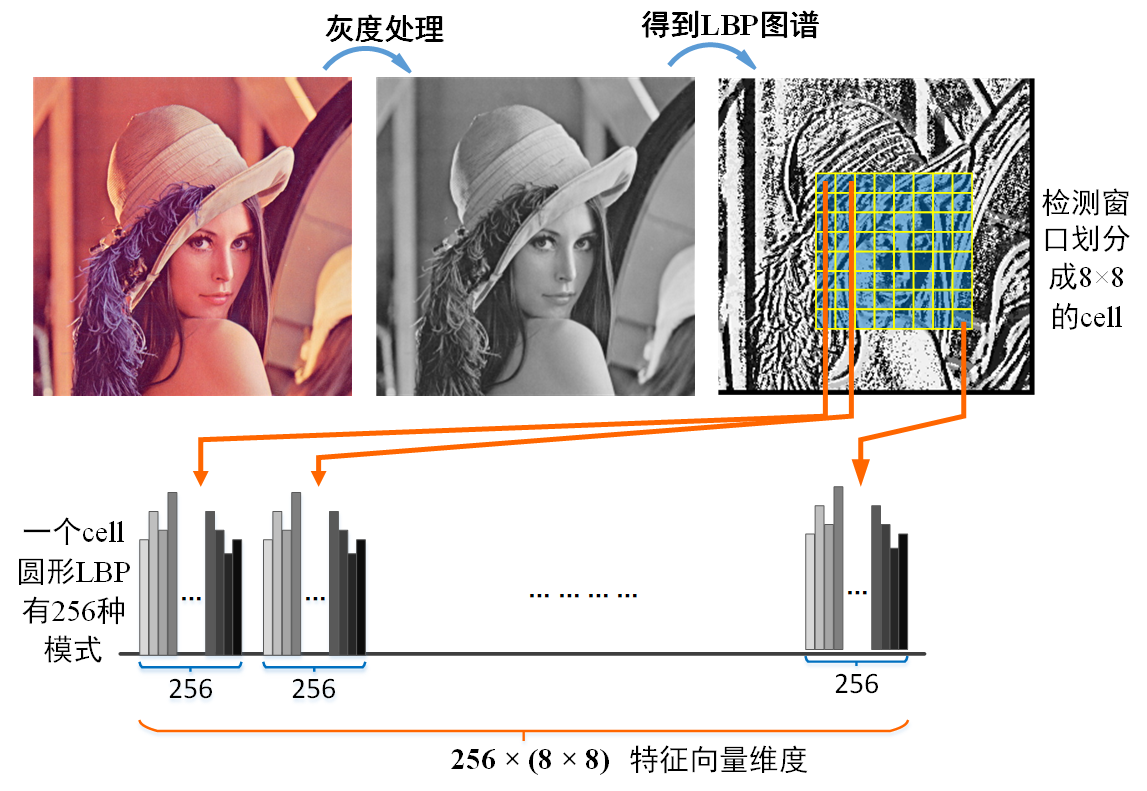采样点为8个，如果用的是原始的LBP或Extended LBP特征，其LBP特征值的模式为256种，则一幅图像的LBP特征向量维度为：64*256=16384维， 而如果使用的UniformPatternLBP特征，其LBP值的模式为59种，其特征向量维度为：64*59=3776维，可以看出，使用等价模式特征，其特征向量的维度大大减少， 这意味着使用机器学习方法进行学习的时间将大大减少，而性能上没有受到很大影响。

...

### 参考

LBP特征原理及代码实现

展开全文• 针对局部二值模式采样不充分和对随机噪声及非一致性光照敏感的问题, 提出一种改进梯度局部二值模式(IGLBP)的人脸描述方法。利用多半径和多方向的采样方式获取两组3 pixel×3 pixel的子邻域, 其由2个半径8个方向的16...
• 首先对待测图像利用基于中心对称像素的局部二值模式算子（C-LBP）定位其特征提取区域，随后基于局部区域中像素之间的关系提出一种改进型的局部二值模式算子，并将其直方图统计作为最终的检测特征。实验证明，与其他...中值滤波 滤波检测
• 然后提取人脸及关键区域的异或-非对称方向性局部二值模式（XOR-AR-DLBP）直方图特征信息，并根据CM对关键区域直方图信息进行加权级联再与整幅人脸图像的特征信息进行融合；最后用SVM分类器进行表情分类识别。该算法...
• 但是如果你想统图像局部直方图的你就得制作一个掩模图像，并将它作用在这个图像上，就会统计掩模这部分的直方图。 histSize :BIN 的数目。表示的是你需要统计的像素范围。你也可以选择[0-10]范围的像素频率...
• 针对局部二值模式（local binary pattern，LBP）描述信息单一以及对噪声敏感的问题，提出一种多尺度自适应阈值局部值模式（multi-scale adaptive local ternary pattern，MSALTP）编码算法。MSALTP首先将原始图像...
• 求取旋转不变的 LBP 的过程示意图： 此时的LBP算子貌似比较不错，但实际使用发现LBPROT并不具有很好地辨别力，因为随着采样点数的增加，进制模式会急剧增多，会使得数据量过大，直方图过于稀疏，不能很好地反映...计算机视觉 python opencv
• 为有效地检测脑电图（EEG）中的癫痫信号，设计一维局部值模式（1D-LTP）算子提取信号特征，并结合主成分分析（PCA）和极限学习机（ELM）对特征进行分类。通过1DLTP算子计算信号点的顶层模式和底层模式下的特征变换...
• 纹理描述在图像分析和模式分类领域具有...最后，在不同尺度上提取3种有效的局部二值模式联合构造纹理描述，并通过直方图降维。试验结果表明，该特征具有较好的可区分性和有效性，可以有效应用到视觉图像的纹理分类中。纹理特征
• 该方法将原始人脸图像滤波下采样处理成低分辨率图像,将其划分成若干块矩形块图像,对每一块图像进行局部二值模式计算,统计出每一块LBP图谱的直方图,再连接在一起成为这幅图片的最终特征向量。经实验表明,该算法在ORL...
• 一）局部二值模式简介 前面介绍过关于主成分分析法的识别方法，这个方法是选取的整幅图像的主成分分量来作为新的特征，从某种意义上说这是一种基于全局特征的方法。但是一般图像识别中，仅仅使用全局特征是不够...数字图像 matlab 机器学习
• 如果局部二值模式特征与方向梯度直方图结合，则可以在一些集合上十分有效的提升检测效果。它将各个像素与其附近的像素进行比较，并把结果保存为进制数。由于其辨别力强大和计算简单，局部二值模式纹理算子已经在...纹理分类 图像处理
• 原始的LBP算子定义为在3*3的窗口内，以窗口中心像素为阈值，将相邻的8个像素的灰度与其进行比较，若周围像素大于或等于中心像素，则该像素点的位置被标记为1，否则为0。这样，3*3邻域内的8个点经比较可产生8...opencv
• LBP（Local Binary Pattern，局部二值模式）是一种用来描述图像局部纹理特征的算子；它具有旋转不变性和灰度不变性等显著的优点。最早由T. Ojala, M.Pietikäinen,和D.Harwood在1994年提出，用于图像的局部纹理特征...图像特征提取算法
• 下面给出本人的matlab实现及示例结果以作示范（第幅测试为随手截取的网上的图片，由于半径及邻域点数不同、是否对均匀模式归化及归化到何方面可能存在分歧，所以结果并不完全一致，这里只是做个示范。...
•     另外，局部差分符号-幅度变换可以将图像局部纹理结构分解成为两种互补成分，即正负二值模式 CLBP_ S，幅度二值模式 CLBP_ M。所述的三种值编码可以任意组合构成最终的CLBP 直方图，能实现比传统的 LBP 更...计算机视觉 人工智能
• 针对完全局部二值模式（CLBP）存在直方图维数过高和特征冗余，会导致识别速度降低和识别率低的问题，提出基于有判别力的完全局部二值模式（Discriminative completed LBP，disCLBP）的人脸表情识别算法。首先，对...
• 文章目录   图像处理流程，通常包含三个阶段：   （1）low level：被称为图像滤波（预处理）。图像to图像——增强操作（锐化、平滑）、差值操作（变大...  （3）High level：被称为图像理解或者模式识别（识别）图像处理 opencv 计算机视觉
• LBPH(Local Binary PatternsHistograms)局部二进制编码直方图，建立在LBPH基础之上的人脸识别法基本思想如下：首先以每个像素为中心，判断与周围像素灰度大小关系，对其进行进制编码，从而获得整幅图像的LBP编码...脸部识别 opencv LBPH
• 局部二值模式（LBP） LBP（Local Binary Pattern，局部二值模式）是一种用来描述图像局部纹理特征的算子；它具有旋转不变性和灰度不变性等显著的优点。它是首先由T. Ojala, M.Pietik?inen, 和D. Harwood 在1994年...
• 对于维的纹理分析，具有很多潜在的应用。例如，工业表层检查，远程监控，生物制药图像分析等等领域。但是在实际应用时，会存在很多问题。主要的问题在于现实世界中的纹理并不像实验中的那么规整，存在着很多变化，...纹理分类 多分辨率分析
• CV计算机视觉核心02-中阶计算机视觉（求图像的灰度直方图、反色变换、gamma变换、直方图直方图均值化、计算机视觉分为以下四大...、LBP局部二值模式、Haar-like、作业（用一个判别器f(x)来决策输出结果y））......计算机视觉 opencv python
• 基于Gabor特征提取，和局部二值模式LBP的人脸识别
• 辛苦博主 ...LBP（Local Binary Pattern，局部二值模式）是一种用来描述图像局部纹理特征的算子；它具有旋转不变性和灰度不变性等显著的优点。它是首先由T. Ojala, M.Pietikäinen, 和 D. Harwood ...特征描述...